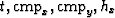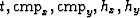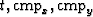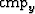# AMO inversion to a common azimuth dataset

Robert G. Clapp

bob@sep.stanford.eda

# ABSTRACT

I cast 3-D data regularization as a least-squares inversion problem. The model space is a four-dimensional () hypercube. An interpolation operator maps to an irregular five dimensional space () which is then mapped back into a four dimensional space by applying Azimuth Move-Out (AMO). A regularization term minimizes the difference between various () cubes by applying a filter that acts along offset. AMO is used to transform the cubes to the same hx before applying the filter. The methodology is made efficient by Fourier-domain implementation and preconditioning of the problem. I apply the methodology on a simple synthetic and to a real marine dataset.

The irregularity of seismic data, particularly 3-D data, in both the model domain (in terms of subsurface position and reflection angle) and the data domain (in terms of midpoint, offset, and time) cause imaging problems. Migration methods desire a greater level of regularity than is often present in seismic surveys. There are two general approaches to deal with this problem. One approach is to treat the imaging problem as an inverse problem. The migration operator can be thought of as a linear transform from the recorded data to image space. Duquet and Marfurt (1999); Prucha et al. (2000); Ronen and Liner (2000) use the migration operator in a linear inverse problem to overcome irregular and limited data coverage. A regularized that encourages consistency over reflection angle is used to stabilize the inverse. These approaches have shown promise but are generally prohibitively expensive, and rely on an accurate subsurface velocity model.

Another approach is to try to regularize the data. AMO provides an effective regularization tool Biondi et al. (1998) and is generally applied as an adjoint to create a more regularized volume. These regularized volumes still often contain in `acquisition footprint' or more subtle amplitude effects. Chemingui (1999) used a log-stretch transform to make the AMO operator stationary in time. He then cast the regularization problem as a frequency-by-frequency inversion problem using a Kirchoff-style AMO operator. He showed that the acquisition footprint could be significantly reduced. The downside of this approach is the relatively high cost of Kirchoff implementation of AMO.

Biondi and Vlad (2001) built on the work of Fomel (2001) and set up an inverse problem relating the irregular input data to a regular model space. They regularized the problem by enforcing consistency between the various () cubes. The consistency took two forms. In the first, a simple difference between two adjacent in-line offset cubes was minimized. In the second, the difference was taken after transforming the cubes to the same offset AMO. Clapp (2005b) set up the data regularization with AMO as an inverse problem creating a full volume ().

In this paper, I modify the approach of Clapp (2005b) so that the model space is a common azimuth volume. I introduce an additional mapping operator that maps from the full () to hy=0 using AMO. In addition, I show that the combination of limited hy and consistent coverage as a function of hx in marine surveys can still produce undesired amplitude variation as a function of. To solve this problem, I introduce an additional regularization term that creates consistency as a function of.